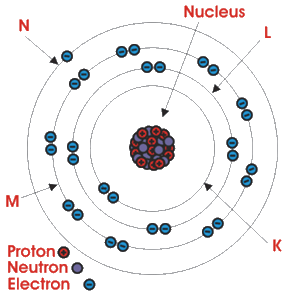Home » » Bohr Model of Atomic Structure

# Atomic Structure

## Bohr Model of Atomic Structure

Most basic unit of any matter is molecule. Molecule is the smallest particle of a matter. A molecule consists of tow or more same or different atoms. Some of us may have misconception that an atom is the smallest particle of an element. Atom is not the smallest physical particle in a matter. The smallest particle of an element does not remain in atomic form it remains in molecular form.

All the properties of a matter persist in its molecules not in atoms. But when tow or more same or different atoms are combined they form the smallest unit of a matter that is molecule. The properties of a molecule depend upon its atomic structure. The properties mean all kind of physical, chemical and electrical properties. So study thebasic structure of atom is very important for analyzing different properties of a matter. As per Bohr model of atomic structure, the mass of an atom and its positive charge are concentrated in a tiny nucleus, while negatively charged electrons revolve around the nucleus in elliptical orbits. The central nucleus contains positively charged protons and neutral neutrons. Hence an atom mainly consists of electrons, protons and neutrons. The table below shows the mass and electric charge of electrons, protons and neutrons.

Particle Mass Charge
Electron9.109 X 10 − 31 kg− 1.602 X 10 − 19 Coulomb
Proton1.672 X 10 − 27 kg+ 1.602 X 10 − 19 Coulomb
Neutron1.675 X 10 − 27 kg0

From above table it is found that a proton is 1840 times heavier than an electron. The absolute value of electric charge of a proton and of an electron are same. So there must be same number of protons and electrons in an electrically neutral atom. The orbits along which the electrons revolve are also known as shells or energy levels. In atomic structure the successive shells are named as K, L, M, N, O, P, and Q as per increasing distance outwards from the nucleus. Each shell or energy level has maximum number of electrons for stability. This maximum number of electron in a shell can be given by the formula 2n2 where n is the shell number in sequential order outward from the nucleus.

As per this formula
the maximum number of electron in first inner shell from nucleus is 2X12 = 2 ,

the maximum number of electron in second inner shell from nucleus is 2X22 = 8 ,

the maximum number of electron in third inner shell from nucleus is 2X32 = 18 ,

and so on.These values are only applicable for inner shell or energy level of anBohr model of atomic structure. For outer most shell of an atom the above rule is not applicable. After fulfilling the maximum numbers of electrons in different inner shells, the rest electrons would be in outer most shell of atom.

Let’s consider one copper atom, where number of electrons is 29. As per this rule the number of electrons in the first, second, third shells that is in K, L and M shell is 2, 8, and 18. The remaining 1 [29 − (2 + 8 + 18) = 1] electron will be in outer most shell i.e. fourth of N shell of the atom.

Every energy level or shell in basic structure of atom can further be sub - divided into different sub-shells or orbitals. The number of sub shells or orbitals in an energy level is equal to its denoting number.
That means the energy level nearest to the nucleus will have one orbital or sub shell as that main shell is denoted as 1 (n = 1).
The next nearer shell from nucleus will have two sub shells or orbitals as this is denoted as 2 (n = 2) .
Similarly third (n = 3) nearer shell from nucleus will have three orbitals and so on.

The orbitals are denoted with s, p, d, f,.............. Among the orbitals of one single shell the first orbital is denoted as s, second orbital is denoted as p, the third one is denoted as d and so on.

So first shell will have one s orbital and is denoted as 1s

The second shell will have one s and one p orbital and they are denoted as 2s & 2p respectively

The third shell will have one s, one p and one d orbital and they are denoted as 3s, 3p & 3d respectively and so on

Here we have another thing to remember that s orbital has one sub orbital and every sub orbital can contains maximum two electrons. The p orbital has 3 sub orbitals and d orbital has 5 sub orbitals. That means p orbital can contain maximum 6(3X2) electrons and d orbital can contain total 10(5X2) electrons.

The lower energy sub orbitals are first filled up then next higher orbital is filled. There would not be any chance of filling up any higher orbitals or sub orbitals, before filling is completed in its lower orbitals.

If we go through the examples below it will be clear to us

Atomic structure of aluminium having 13 electrons

 3p • 3s •• 2p •• •• •• 2s •• 1s ••

Atomic structure of copper having 29 electrons

 3d •• •• •• •• • 4s •• 3p •• •• •• 3s •• 2p •• •• •• 2s •• 1s ••

Here it can be noticed that 3d orbital is in higher energy level than 4s

Atomic structure of silver having 47 electrons

 4d •• •• •• •• • 5s •• 4p •• •• •• 3d •• •• •• •• •• 4s •• 3p •• •• •• 3s •• 2p •• •• •• 2s •• 1s ••

Here it can be noticed that 3d orbital is in higher energy level than 4s similarly 4d orbital is in higher energy level than 5s similarly

--